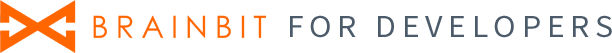# Gain

Package: com.neuromd.neurosdk

An enumeration that is used to obtain the value of the gain of the ADC signal.

Includes the following fields:

 Gain1 The gain is 1 Gain2 The gain is 2 Gain3 The gain is 3 Gain4 The gain is 4 Gain6 The gain is 6. Gain8 The gain is 8 Gain12 The gain is 12